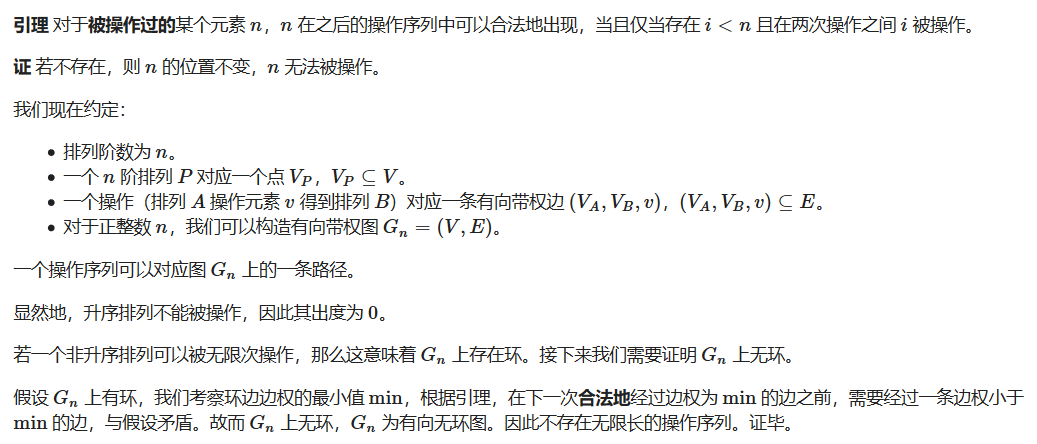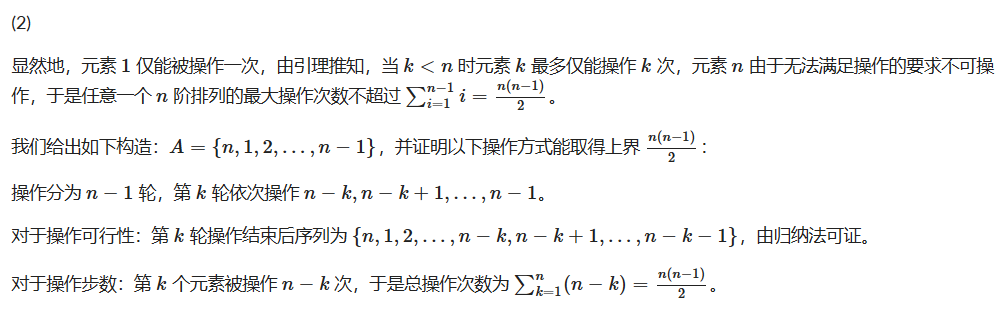## $day1$

T1，你有 $100$张牌，分为红黄蓝三种颜色，每种颜色不超过 $n$张，请问 $n$最多可以等于多少，并且保证你总可以把这些纸牌排成一个 $10$行 $10$列的方阵，使得任何上下相邻或者左右相邻的牌都不相同，并且给出证明。

T2，证明有无穷多质数 $p$，满足 $p|2^{n^3+1}-3^{n^2+1}+5^{n+1}$

T3，找到所有映射 $f$，使得任意 $x,y$，$f(x^2-y^2)=(x-y)(f(x)+f(y))$

T4，给你一个排序算法，每次选取初始排列中的一个比正确位置靠右的数，把这个数移到正确的位置，并且把这中间经过的数都向后移一格，以上称为一次操作，比如 1 5 3 4 2 操作 2 变成 1 2 5 3 4，但是 1 3 4 不能被操作因为不在正确位置的右边。第一问，对于任意一个排列，任意操作，证明经过有限步数会变成顺序排列（即不会出现死循环）。第二问，求这个算法最坏情况下最多要执行的步数。

## day2

T1 就是 $agc018C$。

T2，有一个 $n\leq 20$个点，$m \leq 150$条边的无向图，你可以从任意点出发任意点结束，形成经过 $d \leq 1e9$的一条路径，要求这个路径必须经过前 $k \leq 7$个点，求路径方案数

T3，有一个坐标范围为 $[-10^3,10^3]$的平面直角坐标系，分布了 $100$个瞭望塔，有 $40$个瞭望塔被别人占领了，你在一个点，并且这个点离最近的瞭望塔距离大于 $10m$，你询问了所有瞭望塔离你的距离，但是敌人的瞭望塔会给你一个错误的距离，准确来讲它会随机选一个点告诉你此瞭望塔离那个点的距离，友方的瞭望塔会告诉你正确的距离，但是由于技术原因误差在 $1m$内，求你的坐标，距离是欧几里得距离，坐标都是浮点数。一个数据有 $20$组 $test$

## $day 3$

T1 后来也说是原题，就是 $agc013C$

T2 有 n 个 01 数 $x_i$，在里面选 $n-3$个数并求和=2 的话，称这个算式是一个合法算式，在 $C(n,3)$个算式里挑 $4$个出来，有多少挑法存在一组 $x_i$，使得这四个算式都合法。$n,T \leq 10^5$，$T$是数据组数

$$x_1+x_2+x_3=2$$
$$x_1+x_2+x_4=2$$
$$x_1+x_3+x_4=2$$
$$x_2+x_3+x_4=2$$

T3，在 a 到 b 之间所有数三进制意义下 1 的个数和取模，$70\%$的数据 $a,b\leq 10^{5000}$，$100\%$的数据 $a,b\leq 10^{350000}$

$updates\;on\;2019.9.7$

$$f(1)=k,y=1$$

$$(f(x)-k)(x+1)=(f(x)+k)(x-1)$$

$$f(x)=kx$$